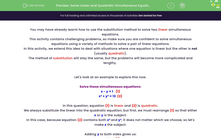# Solve Linear and Quadratic Simultaneous Equations

In this worksheet, students will learn how to solve a pair of simultaneous equations (one linear and one quadratic) using the substitution method.Key stage:  KS 4

Year:  GCSE

GCSE Subjects:   Maths

GCSE Boards:   Pearson Edexcel, OCR, Eduqas, AQA,

Curriculum topic:   Algebra

Curriculum subtopic:   Solving Equations and Inequalities Algebraic Equations

Difficulty level:#### Worksheet Overview

You may have already learnt how to use the substitution method to solve two linear simultaneous equations.

This activity contains challenging problems, so make sure you are confident to solve simultaneous equations using a variety of methods to solve a pair of linear equations.

In this activity, we extend this idea to deal with situations where one equation is linear but the other is not (usually quadratic).

The method of substitution will stay the same, but the problems will become more complicated and lengthy.

Let's look at an example to explore this now.

Solve these simultaneous equations:

x - y = 1   (1)

x² + y² = 13  (2)

In this question, equation (1) is linear and (2) is quadratic.

We always substitute the linear into the quadratic equation, but first, we must rearrange (1) so that either x or y is the subject.

In this case, because equation (2) contains both and y², it does not matter which we choose, so let's make x the subject.

Adding to both sides gives us:

x = 1 + y  (1)

Now we can substitute this into (2):

(1 + y)² + y² = 13  (2)

Now, we need to expand the brackets and simplify:

1 + 2y + y² + y² = 13

2y² + 2y - 12 = 0

This is now a quadratic equation involving y, so needs to be solved using any of the methods we have in our toolkit for solving quadratics.

Firstly we can divide the whole equation by 2 to make life easier:

y² + y - 6 = 0

Always try to factorise before you turn to any other method for solving quadratics, as this is usually the easiest and quickest method.

This equation will factorise into two brackets:

(y + 3)(y - 2) = 0

Either y + 3 = 0 or y - 2 = 0

So y = -3 or y = 2

So we have two possible values for y, and each will have a corresponding value for x.

To find the x-values, always substitute the y-values into the linear equation:

When y = -3  x = 1 + -3 so x = -2

When y = 2  x = 1 + 2 so x = 3

So the two pairs of solutions are x = 3, y = 2 or x = -2, y = -3

These are sometimes written as coordinates: (3 , 2) or (-2 , -3).

At this point, we could check our solutions by substituting them into equation (2).

It will always be the case with these types of simultaneous equation that there will be two pairs of solutions and you need to find them both.

Now, it's time to try some yourself.

The working in some of these questions is long and complex, so make sure you have a pen and paper handy to record your calculations.

You can then compare your working to the working written by our maths teacher.

### What is EdPlace?

We're your National Curriculum aligned online education content provider helping each child succeed in English, maths and science from year 1 to GCSE. With an EdPlace account you’ll be able to track and measure progress, helping each child achieve their best. We build confidence and attainment by personalising each child’s learning at a level that suits them.

Get started